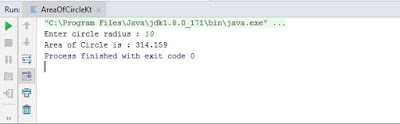# Program to find area of circle in Kotlin

In this article, we will learn simple Kotlin program to find an area of a circle.

Source Code:
fun main(args: Array<String>)
{
if(input.toIntOrNull() != null)
{
val pi: Double=3.14159
print("Area of Circle is : \$area")
}
else
{
}
}
Output:
Area of Circle is : 314.159Program to find an area of a circle in Kotlin - Output

Description:
In the above program, we will take input from a user to know the radius of a circle. To do so we have used readLine() function. Next, we have checked that input accepted from the user is valid integer or decimal number. If an input is not valid then it will print the message to enter valid input.

If the input is a valid integer or decimal number, we will store it to a variable named val radius. Next, we have taken variable pi to hold the value of mathematical constant π, which is 3.14159. To find the area of a circle we have used an equation pi * radius * radius, which is equivalent to πr2.